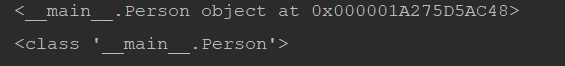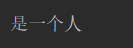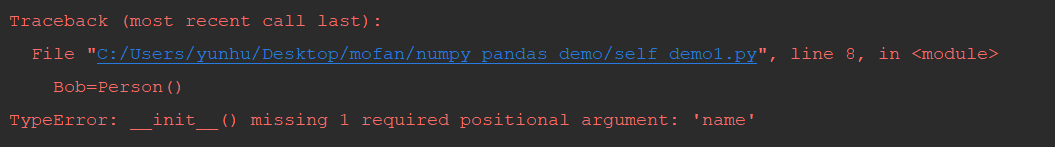# self参数 - __ init__ ()方法 super(Net, self).__init__()是什么

import torch
import torch.nn as nn
import torch.nn.functional as F

class Net(nn.Module):

def __init__(self):
super(Net, self).__init__()
# 输入图像channel：1；输出channel：6；5x5卷积核
self.conv1 = nn.Conv2d(1, 6, 5)
self.conv2 = nn.Conv2d(6, 16, 5)
# an affine operation: y = Wx + b
self.fc1 = nn.Linear(16 * 5 * 5, 120)
self.fc2 = nn.Linear(120, 84)
self.fc3 = nn.Linear(84, 10)

def forward(self, x):
# 2x2 Max pooling
x = F.max_pool2d(F.relu(self.conv1(x)), (2, 2))
# 如果是方阵,则可以只使用一个数字进行定义
x = F.max_pool2d(F.relu(self.conv2(x)), 2)
x = x.view(-1, self.num_flat_features(x))
x = F.relu(self.fc1(x))
x = F.relu(self.fc2(x))
x = self.fc3(x)
return x


• self参数
• __ init__ ()方法
• super(Net, self).init()
接下来就为大家逐一讲解一下。
 def __init__(self):
super(Net, self).__init__()
def forward(self, x):

##### self参数

self指的是实例Instance本身，在Python类中规定，函数的第一个参数是实例对象本身，并且约定俗成，把其名字写为self，也就是说，类中的方法的第一个参数一定要是self，而且不能省略

1. self指的是实例本身，而不是类
2. self可以用this替代，但是不要这么去写
3. 类的方法中的self不可以省略

class Person():
def eat(self):
print(self)

Bob=Person()
Bob.eat()
print(Person)class Person():
def eat(this):
print(this)

Bob=Person()
Bob.eat()
print(Person)##### __ init__ ()方法

class Person():
def __init__(self):
print("是一个人")
def eat(self):
print("要吃饭" )
Bob=Person()class Person():
def __init__(self,name):
print("是一个人")
self.name=name
def eat(self):
print("%s要吃饭" %self.name)

Bob=Person()
Bob.eat()class Person():
def __init__(self,name):
print("是一个人")
self.name=name
def eat(self):
print("%s要吃饭" %self.name)

Bob=Person('Bob')
Bob.eat()class Person():
def __init__(self,name):
print("是一个人")
self.name=name
def eat(self,money):
print("%s要吃饭" %self.name)
self.money=money
def qian(self):
print("花了%s元" %self.money)

Bob=Person('Bob')
Bob.eat(12)
Bob.qian()

##### super(Net, self).init()

python中的super(Net, self).init()是指首先找到Net的父类（比如是类NNet），然后把类Net的对象self转换为类NNet的对象，然后“被转换”的类NNet对象调用自己的init函数，其实简单理解就是子类把父类的__init__()放到自己的__init__()当中，这样子类就有了父类的__init__()的那些东西。

class Net(nn.Module):

def __init__(self):
super(Net, self).__init__()
# 输入图像channel：1；输出channel：6；5x5卷积核
self.conv1 = nn.Conv2d(1, 6, 5)


#!/usr/bin/env python
# -*- coding:utf-8 -*-

class Person(object):
def __init__(self,name,gender,age):
self.name = name
self.gender = gender
self.age = age

class Student(Person):
def __init__(self,name,gender,age,school,score):
#super(Student,self).__init__(name,gender,age)
self.name = name.upper()
self.gender = gender.upper()
self.school = school
self.score = score

s = Student('Alice','female',18,'Middle school',87)
print s.school
print s.name02-243万+
03-013万+
10-152万+
10-0810万+
02-021933
03-09692
08-04906
02-228408
06-27490
08-241万+
11-011万+
08-211万+
07-15354
06-242999
03-041633
07-226019
02-105003
09-20471